# Numbers with the same kernel

Rafael Jakimczuk
Notes on Number Theory and Discrete Mathematics
Print ISSN 1310–5132, Online ISSN 2367–8275
Volume 25, 2019, Number 3, Pages 44—64
DOI: 10.7546/nntdm.2019.25.3.44-64

## Details

### Authors and affiliations

Rafael JakimczukDivision Matematica, Universidad Nacional de Lujan
Buenos Aires, Argentina

### Abstract

In this article we study functions related to numbers which have the same kernel. We apply the results obtained to the sums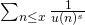, where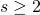is an arbitrary but fixed positive integer and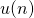denotes the kernel of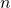. For example, we prove thatwhere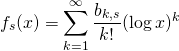and the positive coefficients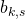of the series have a strong connection with the prime numbers. We also prove thatwhere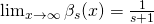. The methods used are very elementary. The case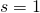, namely, was studied, as it is well-known, by N. G. de Bruijn (1962) and W. Schwarz (1965).

### Keywords

• Kernel function
• Numbers with the same kernel

• 11A99
• 11B99

### References

1. Beukers, F. (1975). The lattice points of n-dimensional tetrahedra, Indag. Math., 37, 365–372.
2. De Koninck, J., Diouf, I., & Doyon, N. (2012). On the truncated kernel function, J. Integer Seq., 15, Article 12.3.2.
3. Hardy, G. H., & Wright, E. M. (1960). An Introduction to the Theory of Numbers, Oxford.
4. Jakimczuk, R. (2008). An observation on the Cipolla’s expansion, Mathematical Sciences. Quarterly Journal, 2, 219–222.
5. Jakimczuk, R. (2007). Integers of the form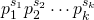, whereare primes fixed, International Journal of Contemporary Mathematical Sciences, 2, 1327–1333.
6. Jakimczuk, R. (2017). On the kernel function, International Mathematical Forum, 12, 693–703.
7. Rey Pastor, J., Pi Calleja, P., & Trejo, C. A. (1969). Analisis Matematico, Volume 1, Kapelusz.

## Cite this paper

Jakimczuk, R. (2019). Numbers with the same kernel. Notes on Number Theory and Discrete Mathematics, 25(3), 44-64, doi: 10.7546/nntdm.2019.25.3.44-64.